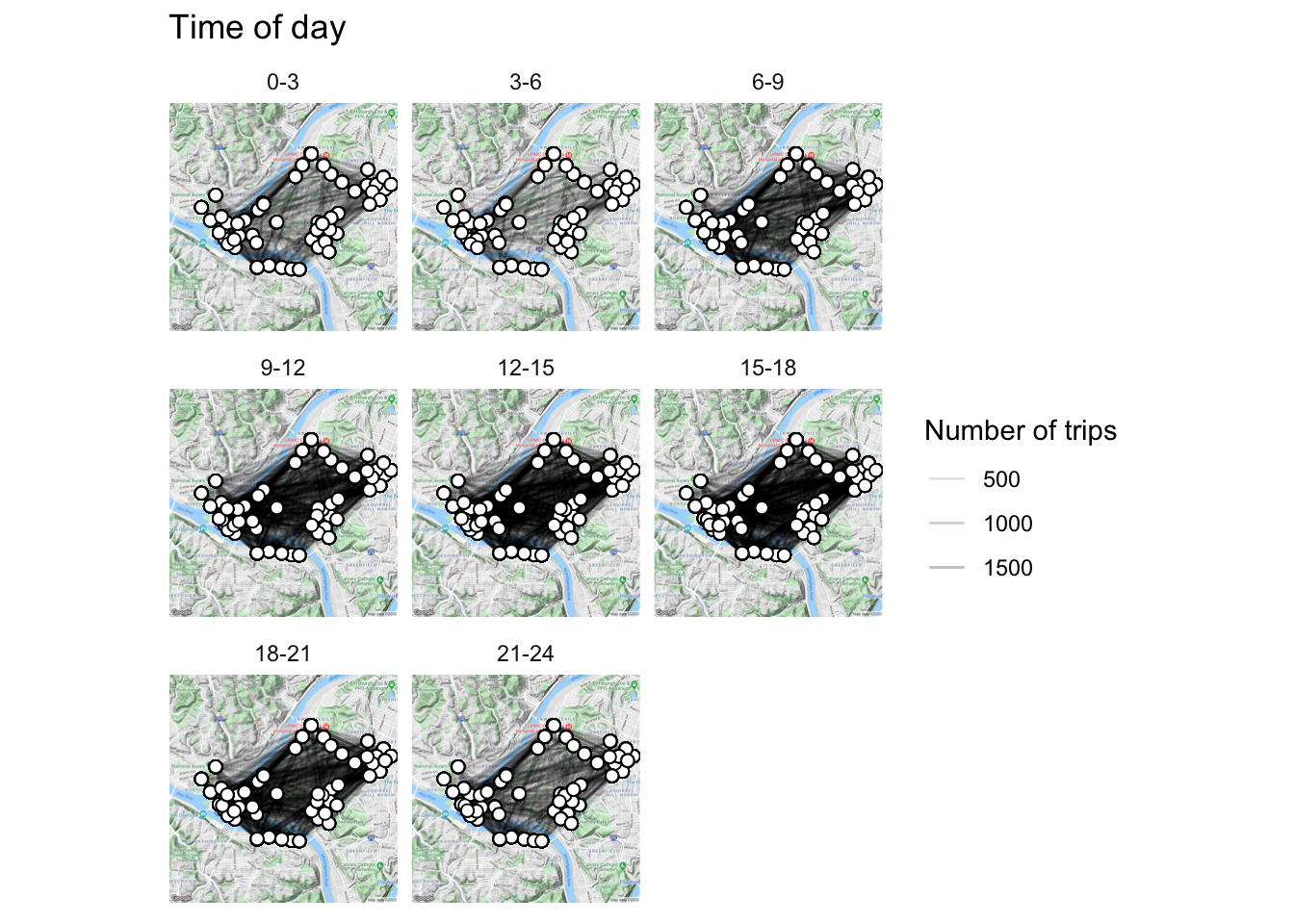# Mapping Healthy Ride Data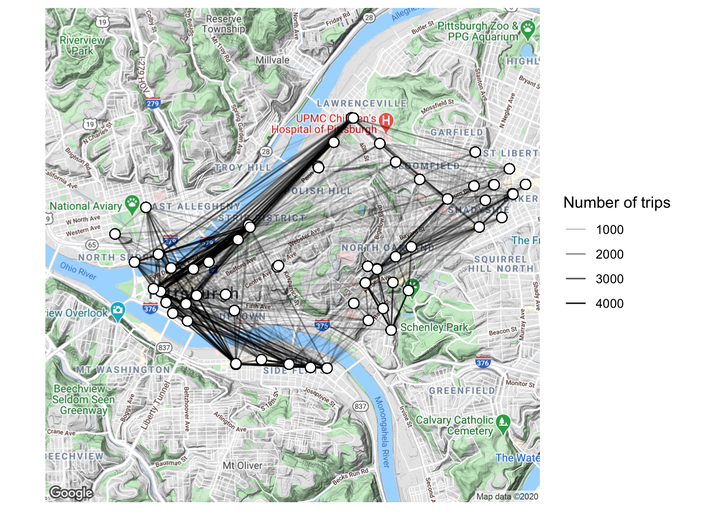This post is about mapping the Healthy Ride dataset in R.

This is my third post about the Healthy Ride bike service in Pittsburgh. You can find the first post and second post on my blog.

First, load the R packages we will be using:

``````library(tidyverse)
library(ggmap)
library(lubridate)
library(viridis)
library(stringr)
library(gghighlight)
library(knitr)
library(kableExtra)``````

Then load the data from the WPRDC (hosted on my GitHub page):

``data <- read_csv("https://raw.githubusercontent.com/conorotompkins/healthy_ride/master/data/data.csv")``

Again, we need to format the data and the column names to make them more useful for analysis. Since this is a repeat of the script from the last post, I will just do it all in one go:

``````colnames(data) <- tolower(colnames(data))
colnames(data) <- gsub(" ", "_", colnames(data))

data_long <- data %>%
rename(start_date_time = starttime,
stop_date_time = stoptime) %>%
gather(date_time_type, date_time, c(start_date_time, stop_date_time)) %>%
select(date_time_type, date_time, everything()) %>%
mutate(date_time_2 = date_time) %>%
separate(date_time, " ", into = c("date", "time")) %>%
mutate(id = row_number(),
date = mdy(date),
year = year(date),
month = month(date, label = TRUE),
week = week(date),
time = hm(time),
hour = hour(time),
wday = wday(date, label = TRUE),
is_weekday = ifelse(wday %in% c("Mon", "Tues", "Wed", "Thurs", "Fri"), "weekday", "weekend"),
yday = yday(date),
mday = mday(date)) %>%
mutate(trip_duration = (tripduration / 60) / 60) %>%
gather(station_id_type, station_id, c(from_station_id, to_station_id)) %>%
gather(station_name_type, station_name, c(from_station_name, to_station_name)) %>%
select(date_time_type,
is_weekday,
date,
year,
month,
hour,
wday,
yday,
mday,
date_time_2,
station_id_type,
station_id,
station_name_type,
station_name,
everything(),
-time)

data_long[1:10, 1:5] %>%
kable("html") %>%
kable_styling(bootstrap_options = c("striped", "hover", "condensed", "responsive"))``````
date_time_type is_weekday date year month
start_date_time weekend 2015-05-31 2015 May
start_date_time weekend 2015-05-31 2015 May
start_date_time weekend 2015-05-31 2015 May
start_date_time weekend 2015-05-31 2015 May
start_date_time weekend 2015-05-31 2015 May
start_date_time weekend 2015-05-31 2015 May
start_date_time weekend 2015-05-31 2015 May
start_date_time weekend 2015-05-31 2015 May
start_date_time weekend 2015-05-31 2015 May
start_date_time weekend 2015-05-31 2015 May

Importantly, we will be excluding trips where the rider started and ended their trip at the same station. The data lacks the granularity to analyze rider location beyond the points where they began and ended their trip.

``````data_long <- data_long %>%
spread(station_name_type, station_name) %>%
filter(from_station_name != to_station_name) %>%
gather(station_name_type, station_name, c(from_station_name, to_station_name))

data_long[1:10, 1:5] %>%
kable("html") %>%
kable_styling(bootstrap_options = c("striped", "hover", "condensed", "responsive"))``````
date_time_type is_weekday date year month
start_date_time weekend 2015-05-31 2015 May
start_date_time weekend 2015-05-31 2015 May
start_date_time weekend 2015-05-31 2015 May
start_date_time weekend 2015-05-31 2015 May
start_date_time weekend 2015-05-31 2015 May
start_date_time weekend 2015-05-31 2015 May
start_date_time weekend 2015-05-31 2015 May
start_date_time weekend 2015-05-31 2015 May
start_date_time weekend 2015-05-31 2015 May
start_date_time weekend 2015-05-31 2015 May

We also need to load the CSV with the longitude and latitude for the Healthy Ride stations

``data_station_locations <- read_csv("https://raw.githubusercontent.com/conorotompkins/healthy_ride/master/data/stations/station_locations.csv")``
``````df_station_totals <- data_long %>%
group_by(station_name) %>%
summarize(number_of_trips = n()) %>%
arrange(desc(number_of_trips), station_name) %>%
left_join(data_station_locations) %>%
select(station_name, number_of_trips, longitude, latitude)

df_station_totals %>%
head() %>%
kable("html") %>%
kable_styling(bootstrap_options = c("striped", "hover", "condensed", "responsive"))``````
station_name number_of_trips longitude latitude
Forbes Ave & Market Square 51924 -80.00308 40.44088
21st St & Penn Ave 47700 -79.98354 40.45212
21st St & Penn Ave 47700 -79.98322 40.45174
Liberty Ave & Stanwix St 45692 -80.00468 40.44133
10th St & Penn Ave (David L. Lawrence Convention Center) 42868 -79.99580 40.44467
S 27th St & Sidney St. (Southside Works) 37356 -79.96611 40.42790

Where are the Healthy Ride Stations?

``````pgh_map <- get_map(c(lon = -79.973859, lat = 40.447095), zoom = 13)
pgh_map <- ggmap(pgh_map)

pgh_map +
geom_point(data = df_station_totals, aes(longitude, latitude, size = number_of_trips),
alpha = .75) +
scale_size_continuous("Number of trips", range = c(.1, 5)) +
theme_minimal() +
theme(axis.text = element_blank(),
axis.title = element_blank())``````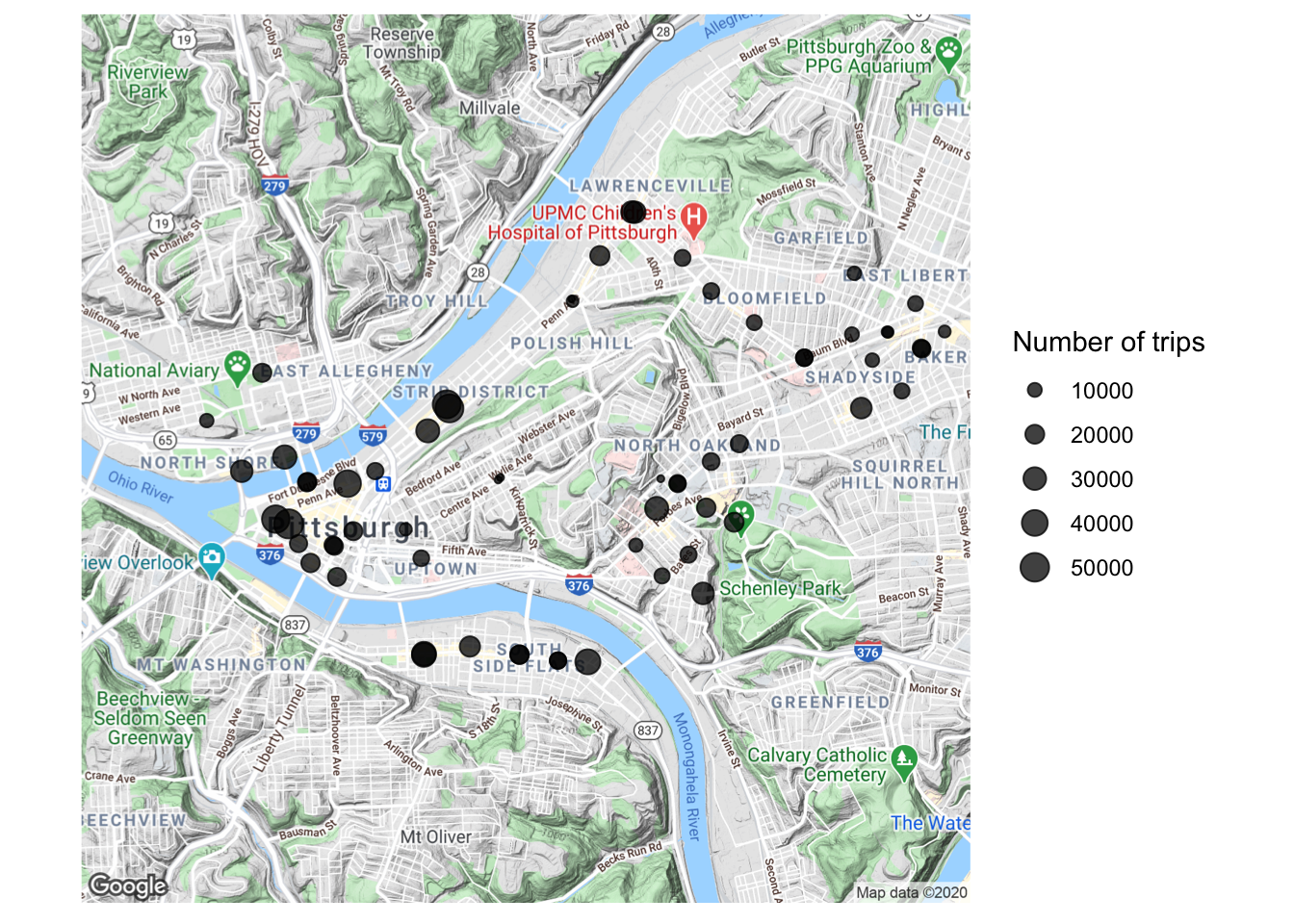Next, join the two dataframes:

``````df_long <- data_long %>%
select(station_name, station_name_type) %>%
group_by(station_name, station_name_type) %>%
summarize(number_of_trips = n()) %>%
arrange(desc(number_of_trips)) %>%
left_join(data_station_locations) %>%
ungroup()

df_long %>%
head() %>%
kable("html") %>%
kable_styling(bootstrap_options = c("striped", "hover", "condensed", "responsive"))``````
station_name station_name_type number_of_trips station_number number_of_racks latitude longitude
Forbes Ave & Market Square to_station_name 29040 1001 19 40.44088 -80.00308
21st St & Penn Ave to_station_name 25748 1017 19 40.45212 -79.98354
21st St & Penn Ave to_station_name 25748 1017 18 40.45174 -79.98322
Liberty Ave & Stanwix St to_station_name 25380 1000 16 40.44133 -80.00468
Forbes Ave & Market Square from_station_name 22884 1001 19 40.44088 -80.00308
10th St & Penn Ave (David L. Lawrence Convention Center) to_station_name 22040 1010 15 40.44467 -79.99580

Do some stations function more as starting points or ending points for trips?

``````pgh_map +
geom_point(data = df_long, aes(longitude, latitude, size = number_of_trips, color = station_name_type),
alpha = .75) +
scale_size_continuous("Number of trips",range = c(.1, 5)) +
scale_color_discrete("Station type") +
facet_wrap(~station_name_type) +
theme_minimal() +
theme(axis.text = element_blank(),
axis.title = element_blank())``````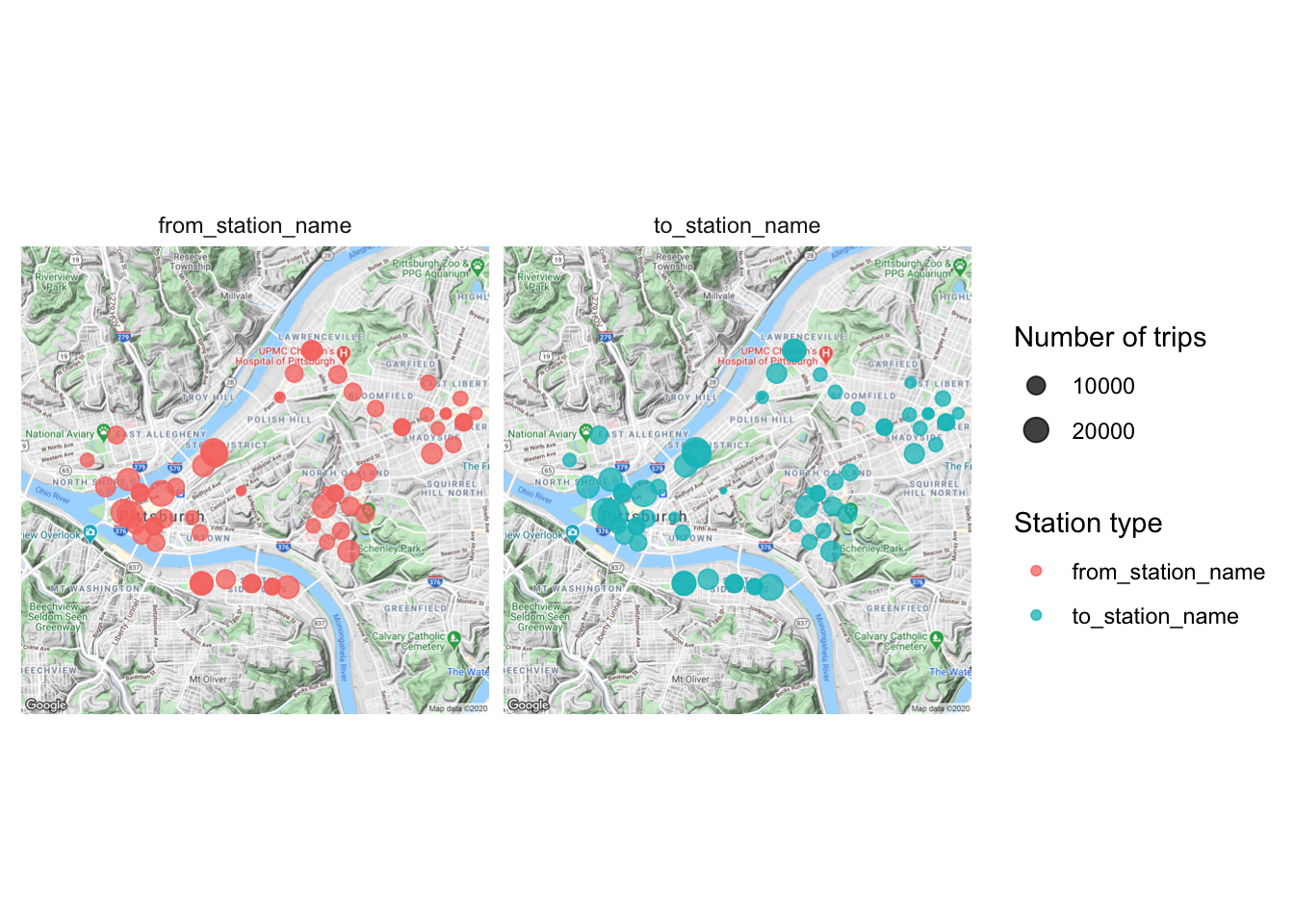No differences are discernible in this view.

A scatter plot shows the differences more effectively:

``````df_from_to <- df_long %>%
spread(station_name_type, number_of_trips) %>%
rename(from_trips = from_station_name,
to_trips = to_station_name) %>%
select(station_name, from_trips, to_trips) %>%
mutate(differential = abs(from_trips - to_trips))

df_from_to %>%
ggplot(aes(from_trips, to_trips)) +
geom_point(size = 1) +
gghighlight(label_key = station_name,
differential > 4000) +
scale_x_continuous(limits = c(0, 30000)) +
scale_y_continuous(limits = c(0, 30000)) +
coord_equal() +
geom_abline() +
labs(x = "From trips",
y = "To trips") +
theme_bw()``````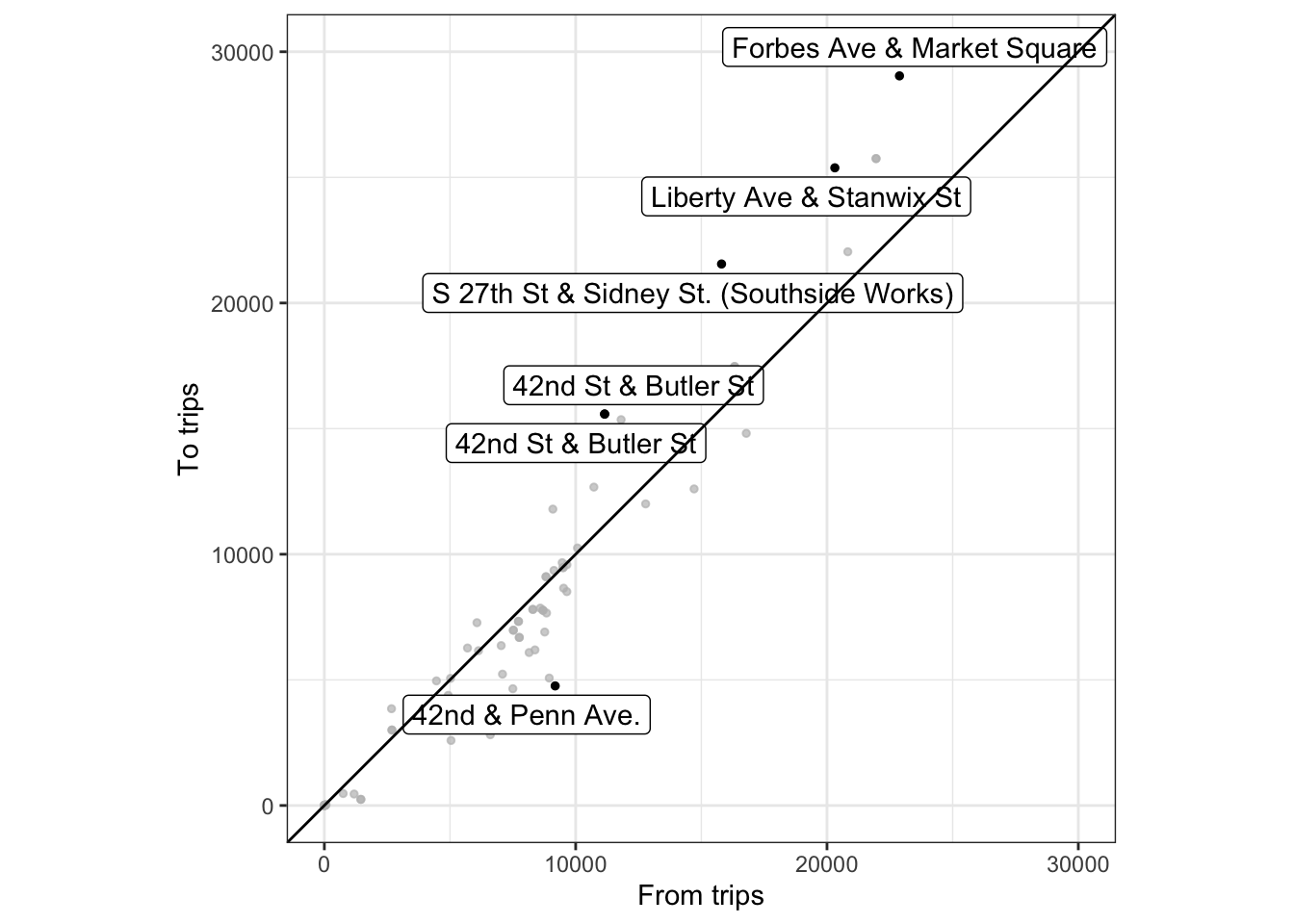What are the top 10 stations in terms of absolute difference between departures and arrivals?

``````df_from_to %>%
ungroup() %>%
arrange(desc(differential)) %>%
top_n(10, differential) %>%
kable("html") %>%
kable_styling(bootstrap_options = c("striped", "hover", "condensed", "responsive"))``````
station_name from_trips to_trips differential
Forbes Ave & Market Square 22884 29040 6156
S 27th St & Sidney St. (Southside Works) 15804 21552 5748
Liberty Ave & Stanwix St 20312 25380 5068
42nd St & Butler St 11152 15580 4428
42nd St & Butler St 11152 15580 4428
42nd & Penn Ave. 9184 4760 4424
Taylor St & Liberty Ave 8948 5072 3876
21st St & Penn Ave 21952 25748 3796
21st St & Penn Ave 21952 25748 3796
Penn Ave & N Fairmount St 6604 2816 3788

Let’s map the connections between stations by drawing lines between the stations.

First, widen the data:

``````df_wide <- data_long %>%
spread(station_name_type, station_name) %>%
select(from_station_name, to_station_name) %>%
left_join(data_station_locations, by = c("from_station_name" = "station_name")) %>%
rename(from_latitude = latitude,
from_longitude = longitude) %>%
left_join(data_station_locations, by = c("to_station_name" = "station_name")) %>%
rename(to_latitude = latitude,
to_longitude = longitude) %>%
group_by(from_station_name, to_station_name, from_longitude, from_latitude, to_longitude, to_latitude) %>%
summarise(number_of_trips = n()) %>%
arrange(desc(number_of_trips)) %>%
mutate(from_station_type = ifelse(from_station_name == to_station_name,
"Same station", "Different station"))``````
``## `summarise()` regrouping output by 'from_station_name', 'to_station_name', 'from_longitude', 'from_latitude', 'to_longitude' (override with `.groups` argument)``
``````df_wide %>%
head() %>%
kable("html") %>%
kable_styling(bootstrap_options = c("striped", "hover", "condensed", "responsive"))``````
from_station_name to_station_name from_longitude from_latitude to_longitude to_latitude number_of_trips from_station_type
10th St & Penn Ave (David L. Lawrence Convention Center) 21st St & Penn Ave -79.99580 40.44467 -79.98354 40.45212 4432 Different station
10th St & Penn Ave (David L. Lawrence Convention Center) 21st St & Penn Ave -79.99580 40.44467 -79.98322 40.45174 4432 Different station
Boulevard of the Allies & Parkview Ave Fifth Ave & S Bouquet St -79.95188 40.43434 -79.95760 40.44232 3888 Different station
21st St & Penn Ave 10th St & Penn Ave (David L. Lawrence Convention Center) -79.98354 40.45212 -79.99580 40.44467 3640 Different station
21st St & Penn Ave 10th St & Penn Ave (David L. Lawrence Convention Center) -79.98322 40.45174 -79.99580 40.44467 3640 Different station
Fifth Ave & S Bouquet St Boulevard of the Allies & Parkview Ave -79.95760 40.44232 -79.95188 40.43434 3560 Different station

Then, layer the data over the map:

``````pgh_map +
geom_segment(data = df_wide, aes(x = from_longitude, xend = to_longitude,
y = from_latitude, yend = to_latitude,
alpha = number_of_trips)) +
geom_point(data = df_wide, aes(from_longitude, from_latitude), shape = 21, size = 3, fill = "white") +
geom_point(data = df_wide, aes(to_longitude, to_latitude), shape = 21, size = 3, fill = "white") +
scale_alpha_continuous("Number of trips", range = c(.0001, 1)) +
theme_minimal() +
theme(axis.text = element_blank(),
axis.title = element_blank())``````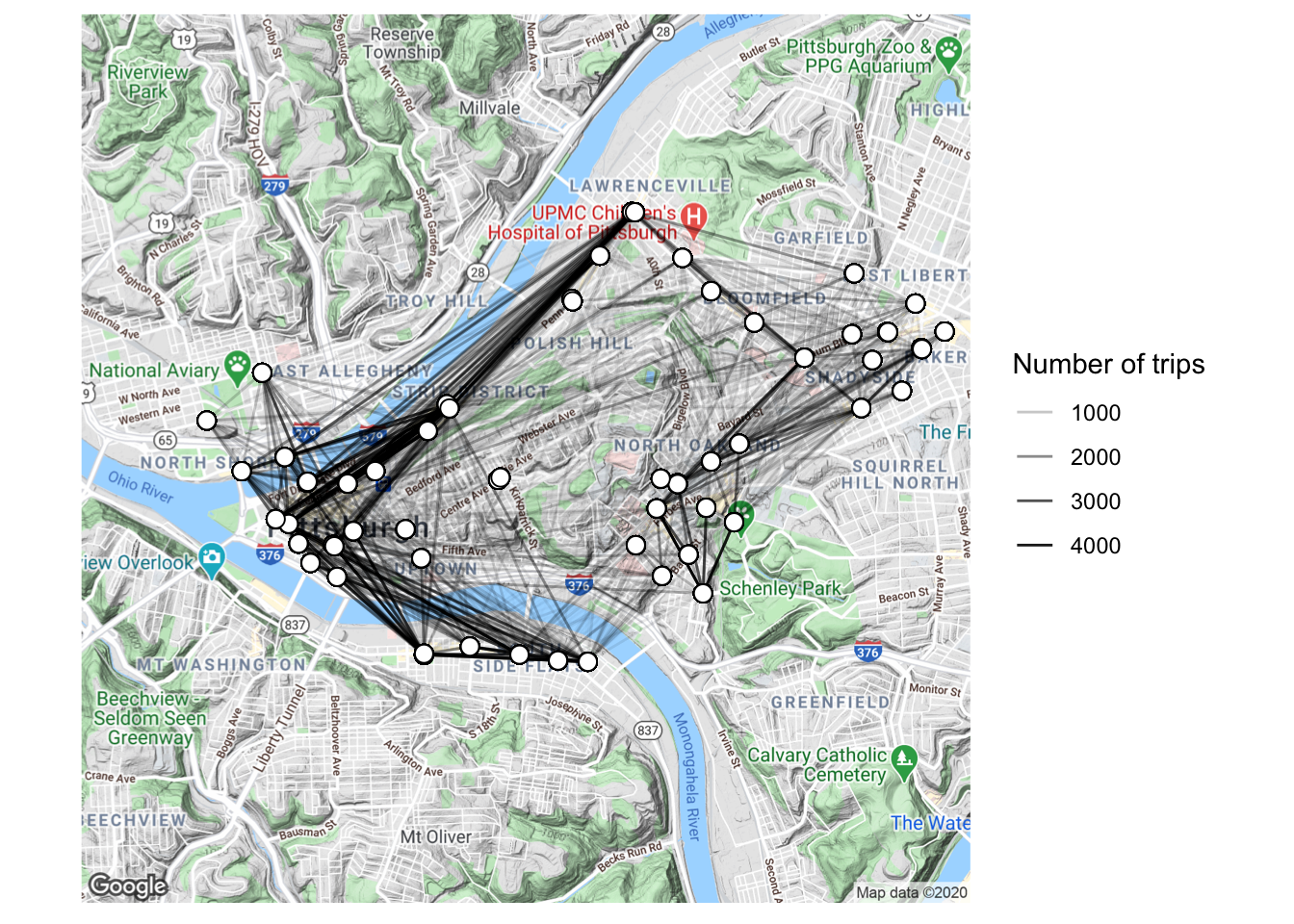We can also facet by the from_station_name variable to see where trips originating from certain stations end at. This plot shows the top 6 stations in terms of trips that began from that station:

``````top_from_stations <- df_wide %>%
group_by(from_station_name) %>%
summarize(number_of_trips = sum(number_of_trips)) %>%
arrange(desc(number_of_trips)) %>%
top_n(6) %>%
select(from_station_name) %>%
unlist()

df_wide_specific_station <- df_wide %>%
filter(from_station_name %in% top_from_stations)

pgh_map +
geom_segment(data = df_wide_specific_station, aes(x = from_longitude, xend = to_longitude,
y = from_latitude, yend = to_latitude,
alpha = number_of_trips), arrow = arrow(length = unit(0.03, "npc"))) +
geom_point(data = df_wide_specific_station, aes(from_longitude, from_latitude),
shape = 1, size = 2) +
geom_point(data = df_wide_specific_station, aes(to_longitude, to_latitude),
shape = 1, size = 2) +
scale_alpha_continuous("Number of trips", range = c(.1, 1)) +
facet_wrap(~from_station_name,
nrow = 2) +
theme_minimal() +
theme(axis.text = element_blank(),
axis.title = element_blank())``````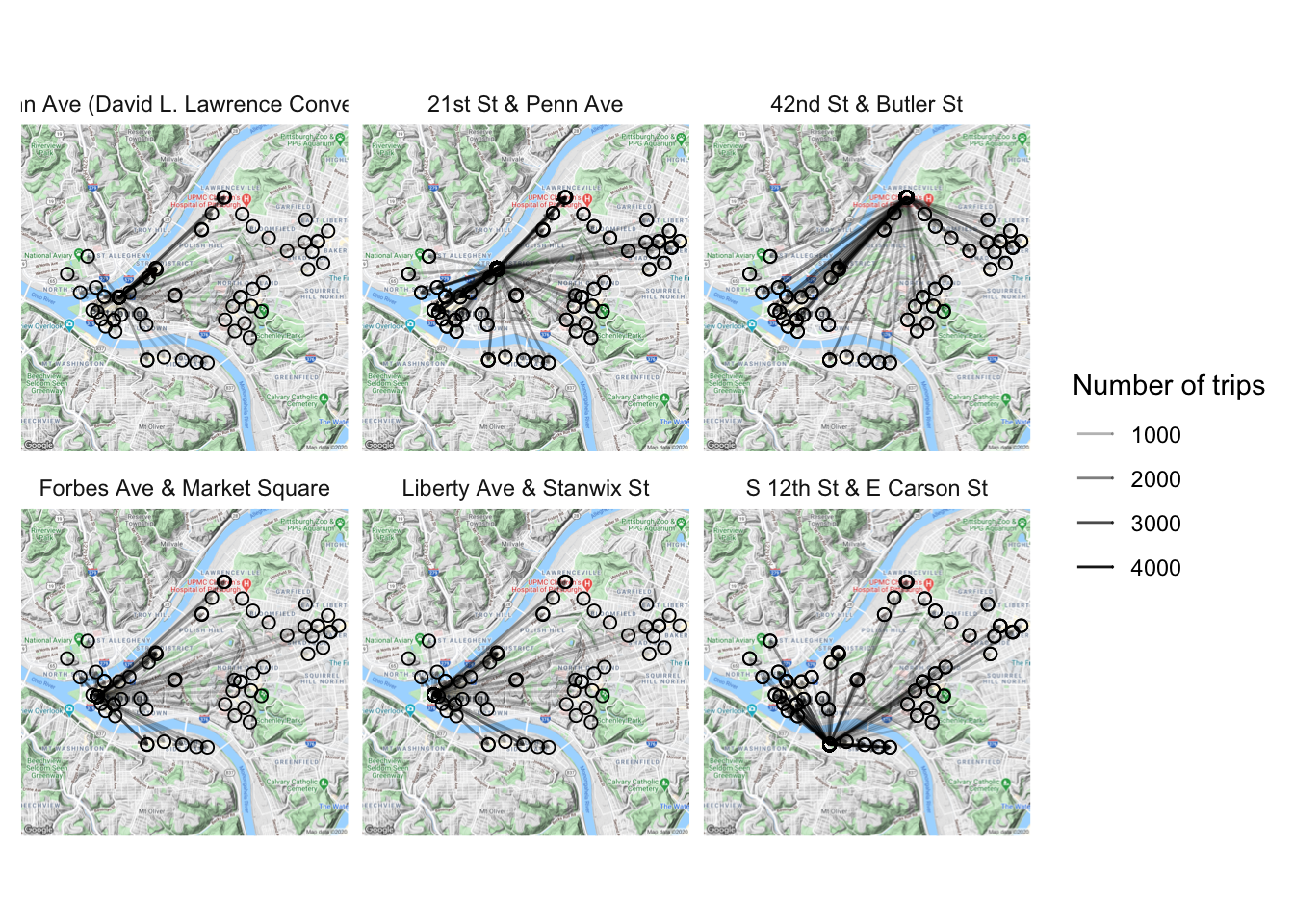We can use the same method to examine the top stations in terms of absolute difference between departing and arriving rides:

``````top_diff_stations <- df_from_to %>%
arrange(desc(differential)) %>%
distinct() %>%
top_n(10) %>%
select(station_name) %>%
unlist()

df_wide_diff_station <- df_wide %>%
filter(from_station_name %in% top_diff_stations)

pgh_map +
geom_point(data = df_wide_diff_station, aes(from_longitude, from_latitude),
shape = 1, size = 2) +
geom_point(data = df_wide_diff_station, aes(to_longitude, to_latitude),
shape = 1, size = 2) +
geom_segment(data = df_wide_diff_station, aes(x = from_longitude, xend = to_longitude,
y = from_latitude, yend = to_latitude,
alpha = number_of_trips),
arrow = arrow(length = unit(0.03, "npc"))) +
scale_alpha_continuous("Number of trips", range = c(.1, 1)) +
facet_wrap(~from_station_name,
nrow = 2) +
theme_minimal() +
theme(axis.text = element_blank(),
axis.title = element_blank())``````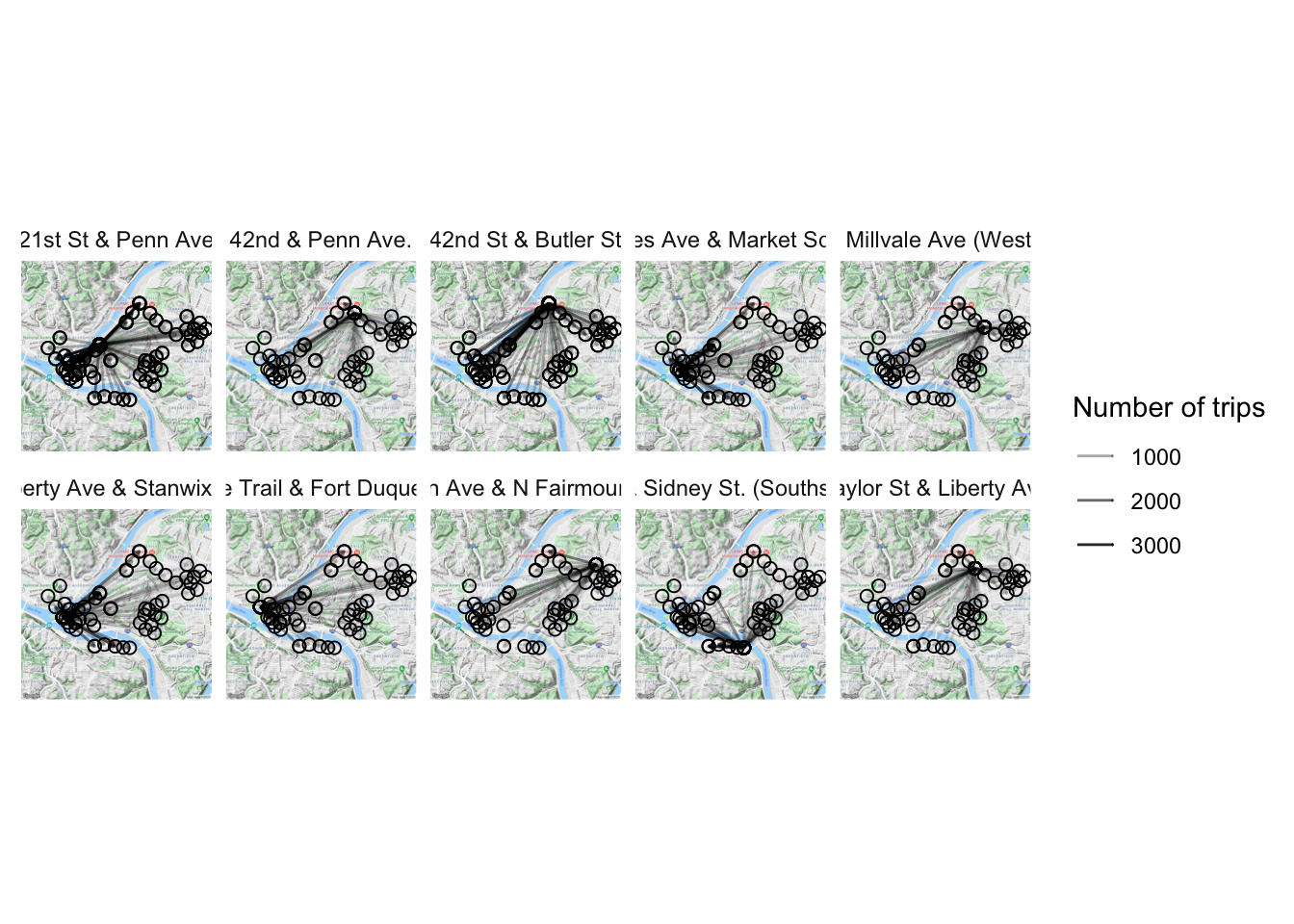There does not appear to be a stark difference in the way the network behaves on weekdays vs. weekends:

``````df_wide_day <- data_long %>%
spread(station_name_type, station_name) %>%
select(from_station_name, to_station_name, is_weekday) %>%
left_join(data_station_locations, by = c("from_station_name" = "station_name")) %>%
rename(from_latitude = latitude,
from_longitude = longitude) %>%
left_join(data_station_locations, by = c("to_station_name" = "station_name")) %>%
rename(to_latitude = latitude,
to_longitude = longitude) %>%
group_by(is_weekday, from_station_name, to_station_name, from_longitude, from_latitude, to_longitude, to_latitude) %>%
summarise(number_of_trips = n()) %>%
arrange(desc(number_of_trips))

pgh_map +
geom_segment(data = df_wide_day, aes(x = from_longitude, xend = to_longitude,
y = from_latitude, yend = to_latitude,
alpha = number_of_trips)) +
geom_point(data = df_wide_day, aes(from_longitude, from_latitude), shape = 21, size = 3, fill = "white") +
geom_point(data = df_wide_day, aes(to_longitude, to_latitude), shape = 21, size = 3, fill = "white") +
scale_alpha_continuous("Number of trips", range = c(.05, .3)) +
facet_wrap(~is_weekday) +
theme_minimal() +
theme(axis.text = element_blank(),
axis.title = element_blank())``````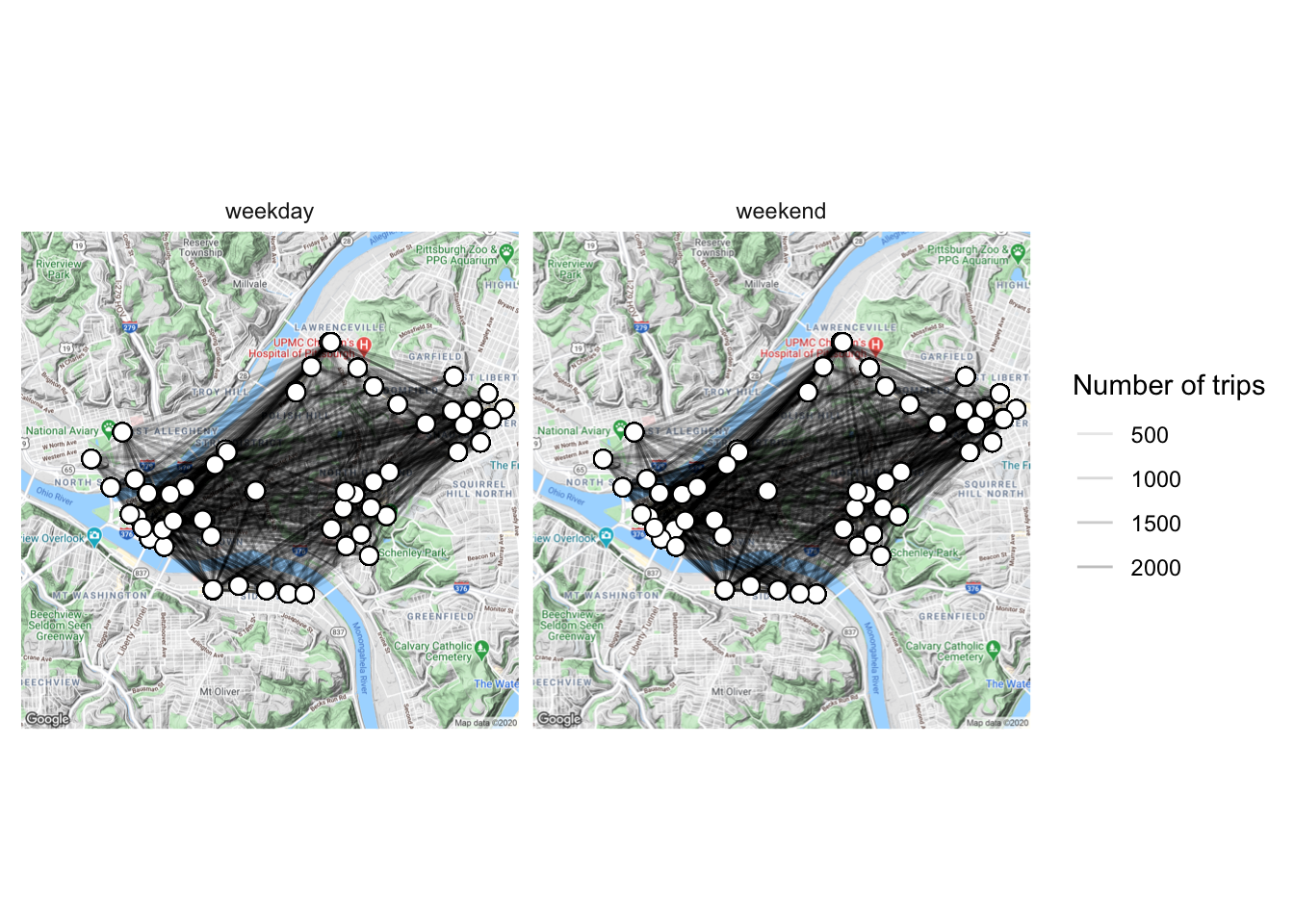There are clear differences in the number of rides across different times of day, but the geographic pattern of departures and arrivals does not appear to change:

``````df_wide_tod <- data_long %>%
spread(station_name_type, station_name) %>%
select(from_station_name, to_station_name, hour) %>%
mutate(time_of_day = cut(hour, breaks = c(-Inf, 3, 6, 9, 12, 15, 18, 21, Inf),
labels = c("0-3", "3-6", "6-9", "9-12", "12-15", "15-18", "18-21", "21-24"),
ordered_result = TRUE)) %>%
left_join(data_station_locations, by = c("from_station_name" = "station_name")) %>%
rename(from_latitude = latitude,
from_longitude = longitude) %>%
left_join(data_station_locations, by = c("to_station_name" = "station_name")) %>%
rename(to_latitude = latitude,
to_longitude = longitude) %>%
group_by(time_of_day, from_station_name, to_station_name,
from_longitude, from_latitude,
to_longitude, to_latitude) %>%
summarise(number_of_trips = n()) %>%
arrange(desc(number_of_trips))

pgh_map +
geom_segment(data = df_wide_tod, aes(x = from_longitude, xend = to_longitude,
y = from_latitude, yend = to_latitude,
alpha = number_of_trips)) +
geom_point(data = df_wide_tod, aes(from_longitude, from_latitude), shape = 21, size = 2, fill = "white") +
geom_point(data = df_wide_tod, aes(to_longitude, to_latitude), shape = 21, size = 2, fill = "white") +
scale_alpha_continuous("Number of trips", range = c(.05, .3)) +
facet_wrap(~time_of_day) +
labs(title = "Time of day") +
theme_minimal() +
theme(axis.text = element_blank(),
axis.title = element_blank())``````